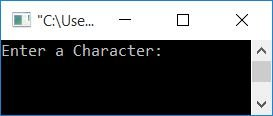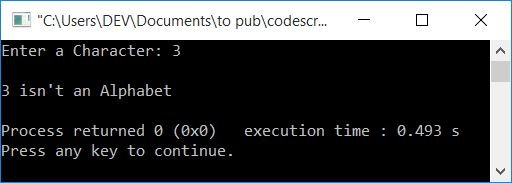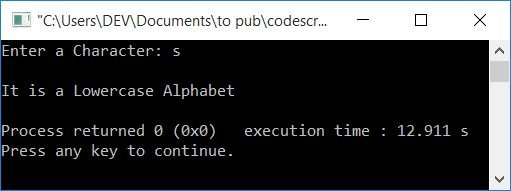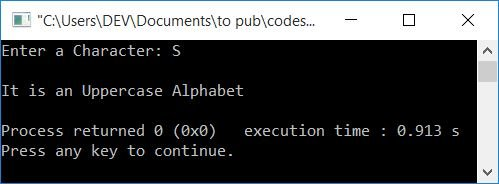# C++ program to check whether the given character is an alphabet or not

In this section, you will learn and receive code for determining whether a given character by the user at run-time is an alphabet or not in C++. The program is created in the following two ways:

## In C++, check whether the character is an alphabet using the character itself

To check whether the entered character is an alphabet or not in C++ programming, you have to ask the user to enter a character and start checking for alphabets.

This program uses an if-else statement to check whether the value of a character is greater than or equal to a and less than or equal to z or not. If this condition evaluates to being true, then it is printed as an alphabet.

Otherwise, check for uppercase. That is, it checks whether the value of a character is greater than or equal to A  (uppercase) and less than or equal to Z (uppercase) or not. If it evaluates to be true, then print it as an alphabet. Otherwise, if both conditions evaluate to false, then print it as not an alphabet.

The following C++ program asks the user to enter a character to check whether it is an alphabet:

```#include<iostream>
using namespace std;
int main()
{
char ch;
cout<<"Enter a Character: ";
cin>>ch;
if((ch>='a' && ch<='z') || (ch>='A' && ch<='Z'))
cout<<endl<<ch<<" is an Alphabet";
else
cout<<endl<<ch<<" isn't an Alphabet";
cout<<endl;
return 0;
}```

This program was built and runs under the Code::Blocks IDE. Here is its sample run:Now supply any input, say "c," and press the ENTER key to check whether "c" is an alphabet or not, as shown in the output given below:Because c is an alphabet, you have seen the above output. Let's try with another input that is a number, say 3. Here is the output you will see for this case:Because 3 is a number and not an alphabet, the program produced the output as shown in the snapshot given above.

If the condition of an if statement evaluates to true, program flow is directed to the if statement's body; otherwise, program flow is directed to else's body.

### In C++, check whether the character is an alphabet using its ASCII value

Now let's create the same program, i.e., to check whether the given or input character is an alphabet or not using its ASCII value. But before going through this program, if you're not aware of the ASCII value of alphabets, then here is the table for you:

Uppercase Alphabet ASCII Value
A 65
B 66
C 67
... ...
Z 90
Lowercase Alphabet ASCII Value
a 97
b 98
c 99
... ...
z 122

Now let's move on and implement it in a program to check for the alphabet or not. The question is, write a program in C++, to receive a character as input and prints whether it is an alphabet or not. The answer to this question is given below:

To check for alphabet using the ASCII value of a character. Then a character is an alphabet if its ASCII value is between 65 and 90 or 97 and 122. Otherwise, it is not an alphabet.

```#include<iostream>
using namespace std;
int main()
{
char ch;
int ascii;
cout<<"Enter a Character: ";
cin>>ch;
ascii = ch;
cout<<endl;
if(ascii>=65 && ascii<=90)
cout<<"It is an Uppercase Alphabet";
else if(ascii>=97 && ascii<=122)
cout<<"It is a Lowercase Alphabet";
else
cout<<"It is not an Alphabet";
cout<<endl;
return 0;
}```

Here is its sample run with user input as s.Here is another sample run with user input as S.Note: The statement "`ascii = ch;`" initializes the ASCII value of the character stored in chto ascii.

#### The same program in different languages

C++ Quiz

« Previous Program Next Program »